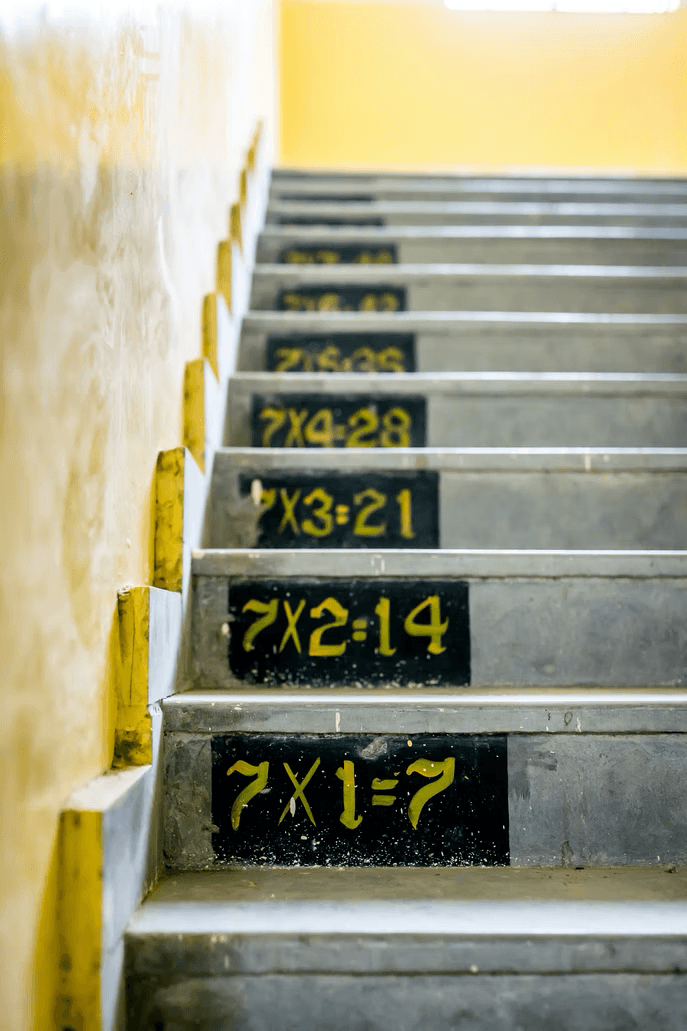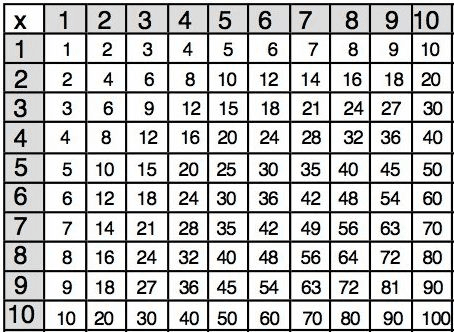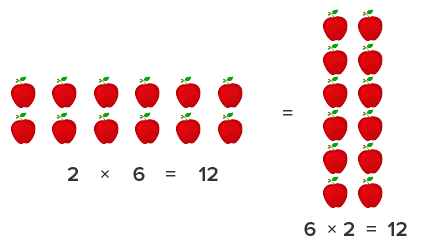# Learn How to Teach Multiplication to Children in a Few Simple Steps

TeachingWe often have to show children how to learn multiplication, but do we truly know how to do that? After addition and subtraction, expansion is the subsequent math concept children have to learn. The idea may seem complicated initially, but children can easily understand it when you teach it well.

## 6 Creative Steps for Teaching Kids Multiplication

There are different ways of teaching multiplication, and each method works differently for different kids. Some children may tackle math problems easily, while others meander around the problem for a while. The goal is to make sure that each kid discovers their preferred method but still learns other techniques, which encourages critical thinking. Here are six steps that show how to teach multiplication to your children or students:The reason why kids think multiplication is complicated is that they have not noticed the patterns yet. Multiplication is addition that is done repeatedly, and when children understand this, they can quickly solve small-scale multiplications. Of course, repeated additions will not work when you have three-digit numbers, but for smaller numbers, it will.

But if this strategy is so simple, how does it work?

If you have the problem 4×2, children can add 4+4 to get 8, which is the same thing. If we are going with a two-digit number and a single-digit one like 10×5, kids can add 10+10+10+10+10 to get 50. The larger the numbers, the higher the repetitive additions involved.

If you need advice on how to teach multiplication facts, repeated addition is one of the easiest ways to teach kids multiplication of small numbers.

### Teach with the multiples of zero

The concept of zero and its lack of value are both exciting and complicated at the same time. For example, your children may find 4+0 =4 interesting, but 4×0 =0 may seem confusing. An easy way to explain this phenomenon will be to use words and illustrations. You can say, “If I give Brandon 4 pencils at no time, how many pencils will Brandon have?” The answer is no pencils, and that is one way you can make children understand the idea of multiplying by 0.

Math for Kids

Is Your Child Struggling With Math?
1:1 Online Math TutoringUsing items that kids like can make your explanation of the multiples of 0 easier to understand. In teaching multiplication, strategies that you can use are numerous; the earlier you find the one that works for your child, the better. Your method of teaching multiplication could include games and activities or a traditional approach.

### Teach multiplying by one

Another part of multiplication that children might find interesting is the process of multiplying by one. Anything multiplied by 1 retains its value, which may be hard for kids to understand since the essence of multiplication is to increase.

In the process of learning multiplication, children are looking for things that will make mathematical and logical sense according to what ‘logic’ means to them at that age. So, when you have taught kids that multiplying means they may have an increased number, you have to explain adequately how multiples of 1 have the same value.

You can use the words method by saying, ‘If I give Brian an orange one time, how many oranges does he have?’ The answer would be one, and that does the trick.

### Move to multiplication tablesAfter you have started with repeated additions and then taught kids the multiples of zero and one, you can change the gear up to the multiplication table. When kids encounter the multiplication table, they know they can repeatedly add numbers after solving the multiples. Start with the 12 tables, and you can increase the tables as this goes. Nevertheless, you must know that kids will start memorizing the multiplication tables instead of solving them because the tables have the results.

One way to make this step an effective learning process for children is to start with the easiest numbers on the table. Print out the multiplication table and hand it to every child. Now, with repeated additions or basic counting, make them solve simple parts of the table. For some children, the multiples of 2, 5, 10, and 11 are the easiest. So, help them tackle more challenging multiplications. Gradually, break down the problems and their solutions until kids understand them.

### Teach the ease of commutative propertiesCommutative property means that the order of numbers does not determine the outcome. 2 can be before 4 in a multiplication table and vice versa, but the answer will always be 8, regardless of the way these numbers are placed. Understanding of commutative properties makes learning multiplication easier for children. Now, they know that 2×13 =26; ergo, if they see 13×2 in another math problem, they would not get confused or scared at the large number in front. Kids could just flip the numbers and solve them easily, which shows the advantage of memorizing the multiplication table.

### Help kids memorize the table

Kids need to have multiplication facts at heart because they would come in handy in real-life situations. The more they remember, the easier it will be to solve more significant multiplication problems in the future. When memorizing the multiplication tables, ask kids to start with the ones they find easy, like the 1s, 2s, 5s, 10s, and 11s before moving to the harder ones.

## Practical approaches to teaching children multiplication

Before you move on to the ways to teach children multiplication, you must first answer the question “when do kids learn multiplication?” Children learn multiplication when they are around three years old; so, your teaching methods and aids must interest them.

Although the best way to teach multiplication to children is the fun way, it is not the only method. There are more straightforward approaches on how to teach kids multiplication, but they may fail when it comes to figuring out how to teach multiplication to struggling students. So, here are five practical ways to teach multiplication to children:

### Play math games

When we were talking about math steps, we mentioned math games a few times, and that is because subtly including math in kids’ playtime is an easy way to learn multiplication. Games are usually a repetitive process that requires to scale through levels, and this repetition helps a child exercise their memories. Therefore, if an activity teaches multiplication lessons, a child will grasp the idea when they play the game repeatedly.

The Timetable Buzz is an activity that all your children can get involved in and enjoy. Pick a family from the multiplication table, and then arrange the children in a line. For example, if you are using the 5s family, every kid should have a number: one says 1, the next one says 2, and then when it gets to 5, yell ‘buzz.’ Move to 6, 7, 8, 9, 10 and ‘buzz!’.

### Use math websites

Yes, math tutors do a great job teaching multiplication strategies, but the best way to learn multiplication is to practice it alone. Introduce math websites to your children and their parents and have them sign up. These websites offer math videos and games that would excite children, teach them, and make your job easier in class. Brighterly is a website that has a 1:1 system of learning for kids. A tutor pays attention to details, helping children learn faster; thus, this source is one of the ways of how to learn multiplication fast.

### Introduce fun math books in the classroom

Colorful math books are a fun way to teach multiplication to kids because such books are captivating. They can hold children’s attention, which can help them gain interest in the subject.

Some math books contain repeated lessons with animals or other colorful items. So, when picking math books, select the ones which would make your kids super excited to open, even when they are not in class. Furthermore, math books will make it easier for you to give homework to your kids because you know they will attempt the problems.

Math for Kids

Is Your Child Struggling With Math?
1:1 Online Math Tutoring### Break the times table into smaller chunks

Teaching your children everything on the 12 tables will be futile. It will leave no space in their little brains to understand the concept. You can, however, start by helping them memorize the table based on individual numbers.

So, pick one number. It’s ideal to start from a small number and help kids master it completely. As children proceed, they will quickly understand how to multiply other numbers. Essentially, don’t try to cram their brains with too much information because it is ineffective.

### Give children space to practice

Children make progress when they don’t feel pressure. So, as a guardian or teacher who is trying to help younglings learn multiplication, you have to give them space to learn without interference. However, ensure you monitor the progress and correct kids when necessary.

Remember that they are kids and are allowed to make mistakes. They will thrive with as much space as possible.

### Recite the multiplication table

Reciting the table is an easy way to learn multiplication. In the description of steps to teach multiplication, we mentioned that children have to memorize the table, and recitation is an excellent way to achieve this. As a parent, you can make kids recite the table on their way to school. If you are a teacher, you can ask kids to recite it before the start of daily activities. Use this easy way to teach multiplication, and the kids will memorize the table in no time.

### Use word examples

We explained this approach earlier in the article. Instead of showing how to solve math problems using numbers, add extra words to make kids talk. Incorporate word problems using storytelling into your multiplication lessons, and ensure children are actively involved. You can use kids’ names for better engagement.

The trick is to make children feel like part of an unfolding multiplication drama. This method will excite them and make your job easier as a caregiver, and that’s how to explain multiplication to a kid.

## Conclusion

Multiplication can be an easy concept if you teach it right. There are many big numbers in multiplication, but kids can learn to work with them as long as they have proper support and learning tools. Common core standards require that children look for patterns in solving math problems, and this is what will work for multiplication.

You can also use multiplication games and storytelling to make the learning process less tedious. Brighterly offers an array of fun activities and games to reduce the stress of learning multiplication. So, children can now learn all the facets of multiplication using unconventional but effective methods.

Math Catch Up Program• Learn Math Simple - Fast - Effective
• Overcome math obstacles and reach new heights with Brighterly.

Simple - Fast - Effective
Overcome math obstacles and reach new heights with Brighterly.